# Easiest colleges to get into in Kansas

Top 10 colleges in Kansas with the highest acceptance rates
What are the easiest colleges to get into in Kansas? We've got you covered. We've compiled a national college database and have created a list of the easiest colleges to get into in Kansas below. These are the easiest 4 year colleges to get into in Kansas that have the highest acceptance rates, and are more likely to accept low GPAs, GEDs, and everything in between. There are tons of good colleges below that accept more applicants, and we also share their average SAT and ACT scores so that you can see where you're most competitive. Read on to find out more.

## Hesston College acceptance rate

Hesston College acceptance rate is 100%.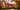The average SAT score for Hesston College is 830.

• SAT composite: 830
• SAT math: 400

The average ACT score for Hesston College is 15.

## Washburn University acceptance rate

Washburn University acceptance rate is 98.8%.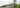The average SAT score for Washburn University is 1110.

• SAT composite: 1110
• SAT math: 555

The average ACT score for Washburn University is 22.

## Wichita State University acceptance rate

Wichita State University acceptance rate is 97.1%.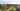The average SAT score for Wichita State University is 1140.

• SAT composite: 1140
• SAT math: 580

The average ACT score for Wichita State University is 23.

## Kansas State University acceptance rate

Kansas State University acceptance rate is 94.6%.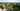The average SAT score for Kansas State University is 1160.

• SAT composite: 1160
• SAT math: 580

The average ACT score for Kansas State University is 24.

## The University of Kansas acceptance rate

The University of Kansas acceptance rate is 93.4%.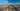The average SAT score for The University of Kansas is 1240.

• SAT composite: 1240
• SAT math: 620

The average ACT score for The University of Kansas is 26.

## Benedictine College acceptance rate

Benedictine College acceptance rate is 92.8%.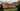The average SAT score for Benedictine College is 1170.

• SAT composite: 1170
• SAT math: 580

The average ACT score for Benedictine College is 25.

## Fort Hays State University acceptance rate

Fort Hays State University acceptance rate is 89%.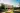The average SAT score for Fort Hays State University is 1080.

• SAT composite: 1080
• SAT math: 540

The average ACT score for Fort Hays State University is 21.

## Pittsburg State University acceptance rate

Pittsburg State University acceptance rate is 87.2%.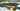The average SAT score for Pittsburg State University is 1110.

• SAT composite: 1110
• SAT math: 555

The average ACT score for Pittsburg State University is 22.

## Emporia State University acceptance rate

Emporia State University acceptance rate is 82.8%.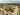The average SAT score for Emporia State University is 1060.

• SAT composite: 1060
• SAT math: 540

The average ACT score for Emporia State University is 22.

## McPherson College acceptance rate

McPherson College acceptance rate is 77.1%.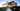The average SAT score for McPherson College is 1090.

• SAT composite: 1090
• SAT math: 550